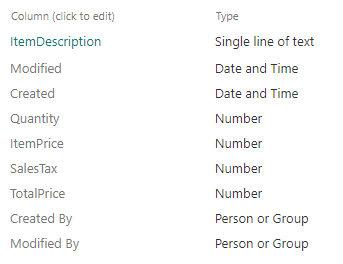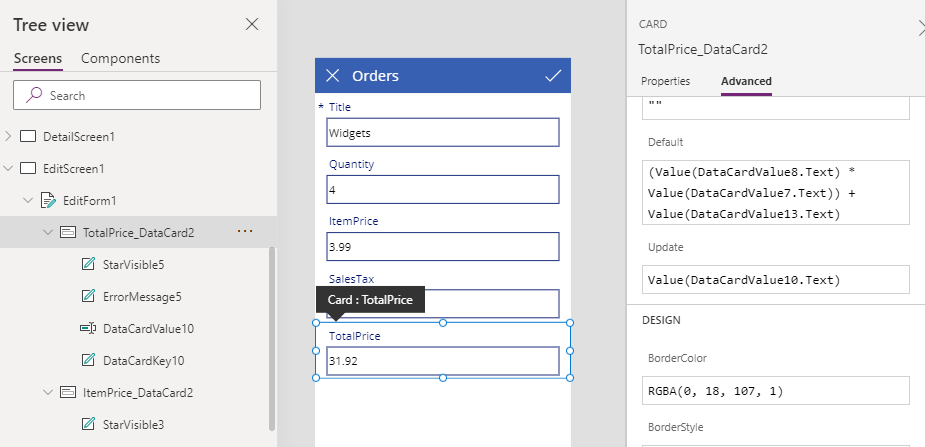# Forms - How to calculate values (eg sums and products) and store the results in SharePoint or other datasource

A simple and basic question that often arises is - how do I perform calculations against fields and store the results in SharePoint or a database? This post walks through how to carry out this task with forms.

A very common requirement is to perform calculations against input values and to store the results in a data source.

There are plenty of ways to carry out this task and a regular occurance I see are from app builders who attempt to perform this task by calling the Patch function. These users can struggle with the necessary syntax and therefore, this post walks through a less complex way to carry out this task by using a form.

## Walkthrough - How to calculate values in a form

To demonstrate, let's take the example of a typical order form. The screenshot beneath shows the fields from our source SharePoint list. These include item description, item price, quantity, sales tax, and total price fields.The example requirement is to create a form that enables the user to enter the item description, price, and quantity. The form then calculates the sales tax and total price. Importantly, it should save these calculated values into the SharePoint list.

## Building a form and defining calculations

The first step is to create an edit form that's based on the SharePoint list.

With this example, we'll assume a sales tax of 20%, and we'll configure the form to calculate sales tax value based on the product of the 'item price' and quantity.

To perform this calcuation, we first unlock the 'sales tax' card. We can then apply the calculation by setting the Default property of the 'sales tax' card to the following formula:

`Value(DataCardValue8.Text) * Value(DataCardValue7.Text) * 0.2`

In this example, the item price and quantity text input controls are called DataCardValue8 and DataCardValue7 respectively, and we can determine these names through the tree view.## Calculating the sums of fields

To calculate the total price, we repeat the same process. The value of of the total price will be the product of the item price and quantity, plus the sales tax.

To perform this calcuation, we unlock the 'total price' card, and we can apply the calculation by setting the Default property of the 'total price' card to the following formula:

`(Value(DataCardValue8.Text) * Value(DataCardValue7.Text)) + Value(DataCardValue13.Text)`

Here, DataCardValue13 is the name of the 'sales tax' text input control.At this stage, we can run the form and enter all the details. The 'sales tax' and 'total price' values will calculate automatically as expected. We can save these calculated values by submitting the form by calling the SubmitForm function.

## Hiding the calculated values and customising the form

To improve the appearance of forms, a common requirement is to hide the calculated values and to retain the ability to save those results to SharePoint or the data source. We can accomplish this by setting the 'Visible' property of the data cards that we want to hide to 'false'

We can further customise the appearance of a form displaying the calculated values in other parts of the app or the screen. We can accoplish this by referencing the hidden text input controls, as highlighted beneath.## Conclusion

A basic and common requirement is to calculate values based on input values and to store those results in SharePoint or a database. This post walked through how to carry out this task with using forms.